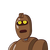# 40.Solve the equation 3x + 4 = 2x – 1, and represent the solutions on(i) the number line(ii) the Cartesian plane​

40.Solve the equation 3x + 4 = 2x – 1, and represent the solutions on
(i) the number line
(ii) the Cartesian plane​

### 1 thought on “40.Solve the equation 3x + 4 = 2x – 1, and represent the solutions on<br />(i) the number line<br />(ii) the Cartesian plane​”

1.Step-by-step explanation:

3x +4 = 2x-1

3x-2x=-4-1

x= -5